# Section 4 1 The Product Quotient and Power

• Slides: 92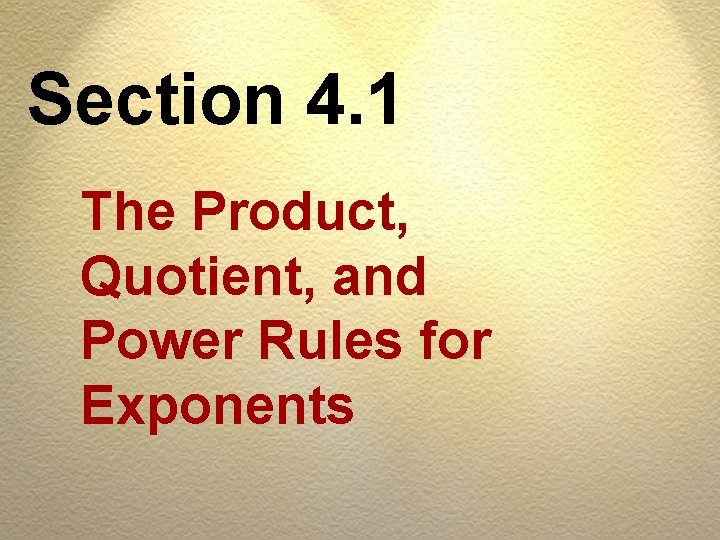Section 4. 1 The Product, Quotient, and Power Rules for Exponents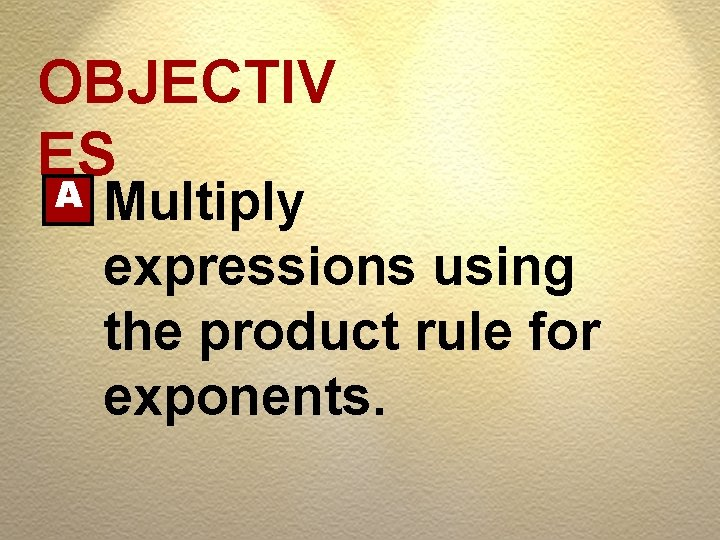OBJECTIV ES A Multiply expressions using the product rule for exponents.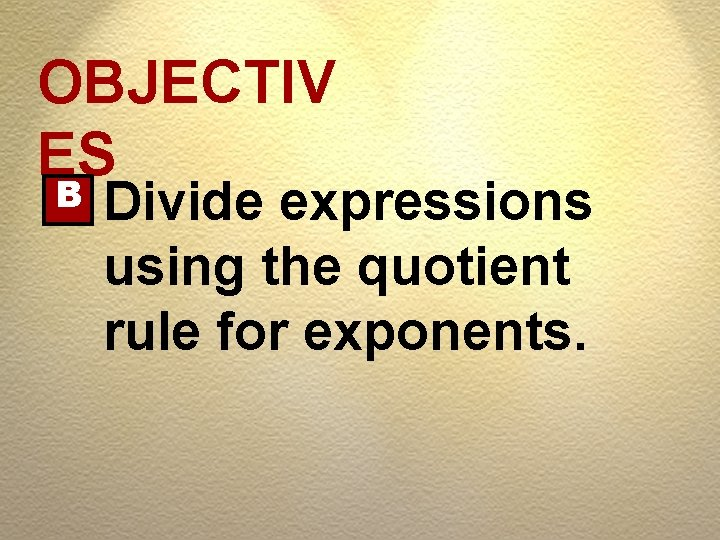OBJECTIV ES B Divide expressions using the quotient rule for exponents.OBJECTIV ES C Use the power rules to simplify expressions.RULES Signs for Multiplication 1. When multiplying two numbers with the same sign, product is positive (+).RULES Signs for Multiplication 2. When multiplying two numbers with different signs, product is negative (-).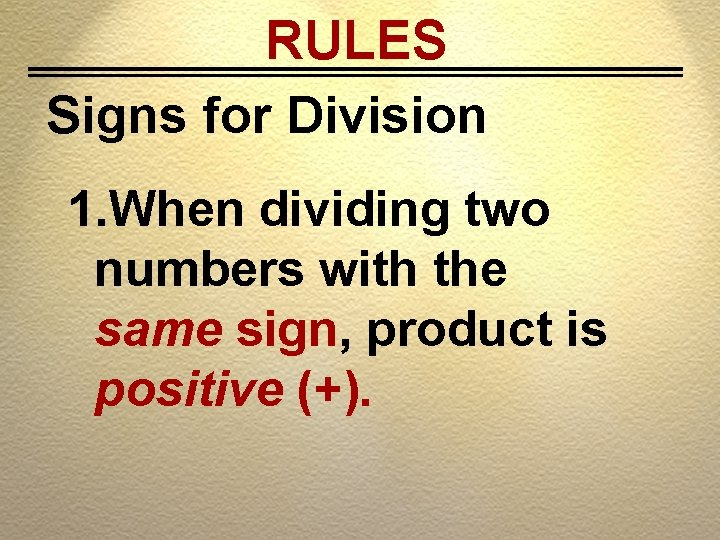RULES Signs for Division 1. When dividing two numbers with the same sign, product is positive (+).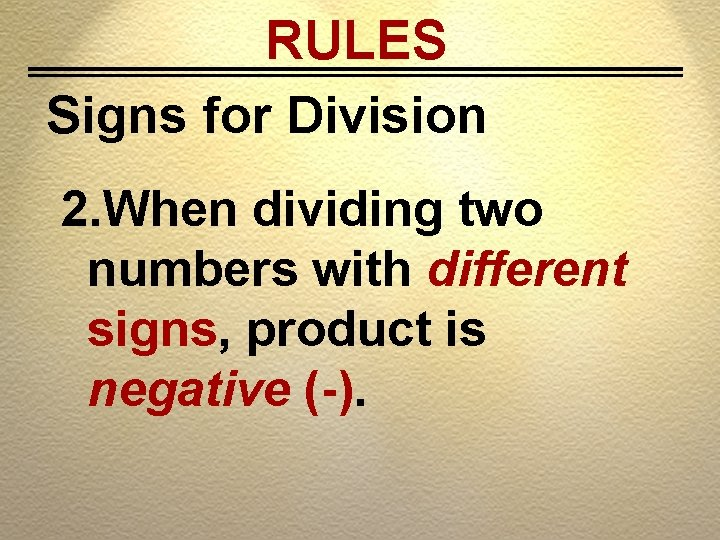RULES Signs for Division 2. When dividing two numbers with different signs, product is negative (-).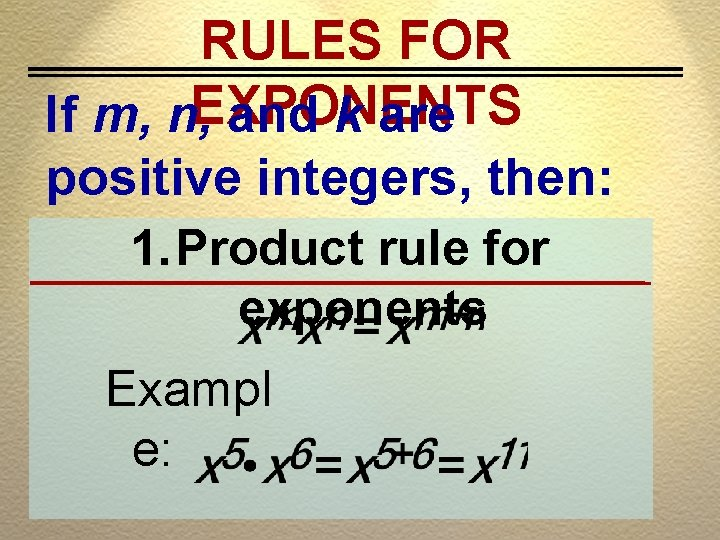RULES FOR If m, n, EXPONENTS and k are positive integers, then: 1. Product rule for exponents Exampl e: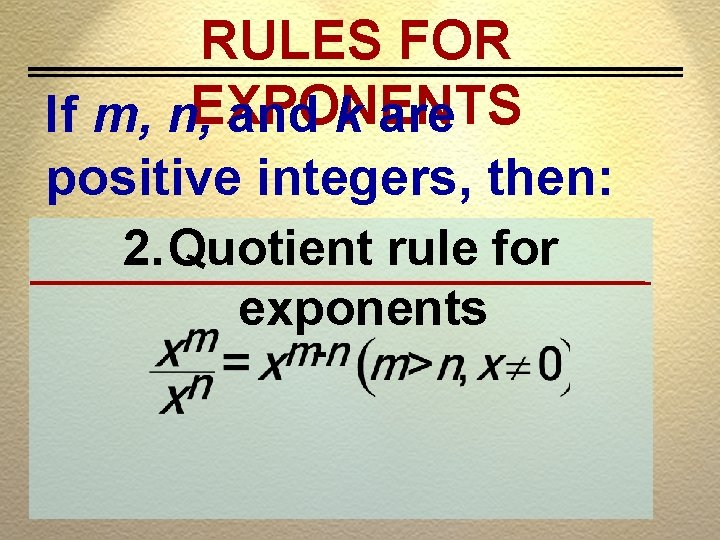RULES FOR If m, n, EXPONENTS and k are positive integers, then: 2. Quotient rule for exponents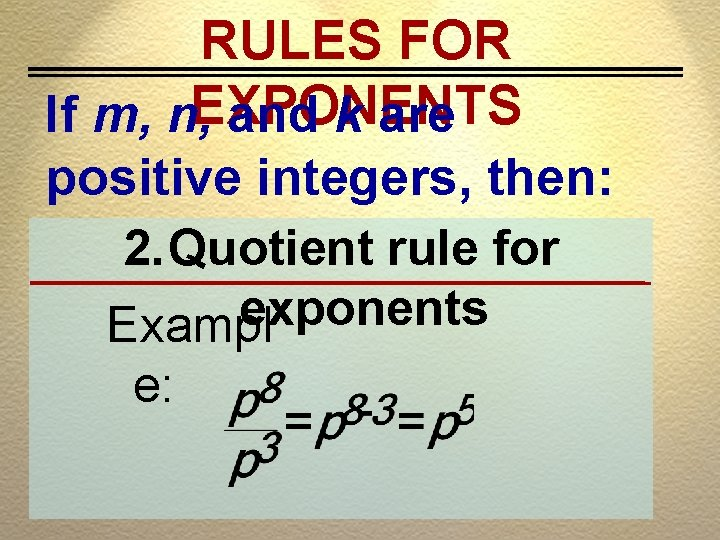RULES FOR If m, n, EXPONENTS and k are positive integers, then: 2. Quotient rule for exponents Exampl e:RULES FOR If m, n, EXPONENTS and k are positive integers, then: 3. Power rule for productsRULES FOR If m, n, EXPONENTS and k are positive integers, then: 3. Power rule for products Example:RULES FOR If m, n, EXPONENTS and k are positive integers, then: 4. Power rule for quotients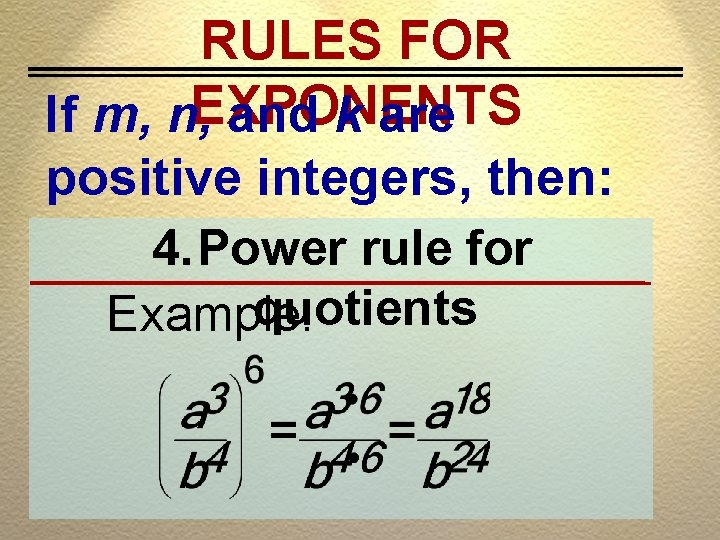RULES FOR If m, n, EXPONENTS and k are positive integers, then: 4. Power rule for quotients Example:Chapter 4 Exponents and Polynomials Section 4. 1 Exercise #1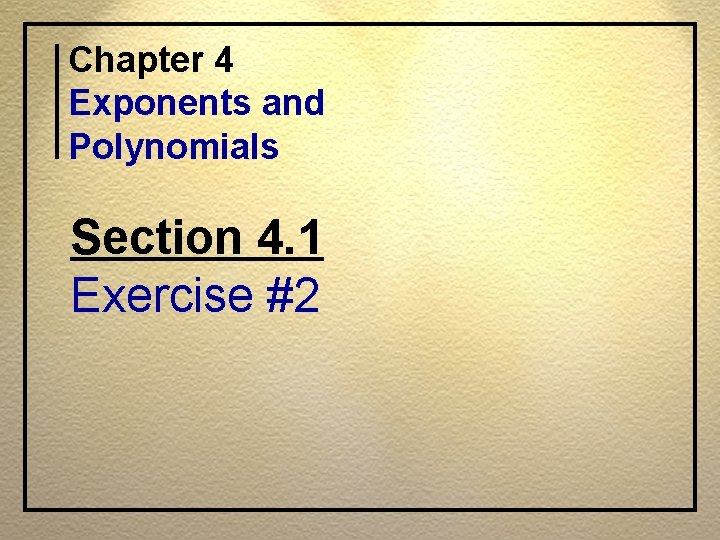Chapter 4 Exponents and Polynomials Section 4. 1 Exercise #2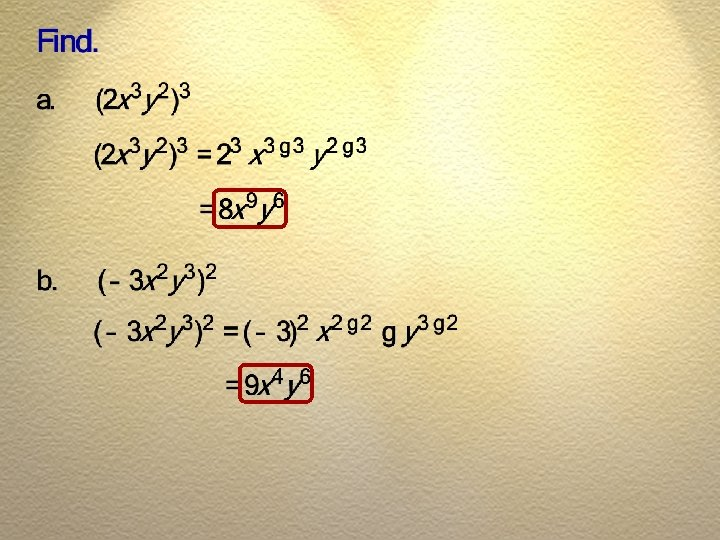Section 4. 2 Integer Exponents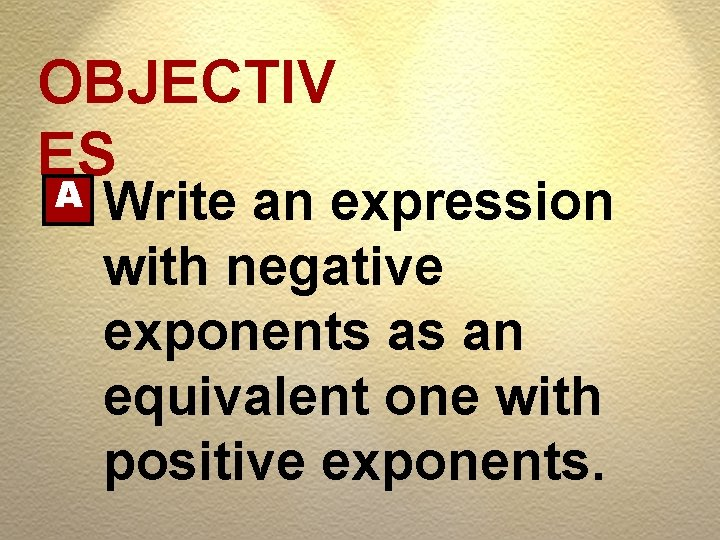OBJECTIV ES A Write an expression with negative exponents as an equivalent one with positive exponents.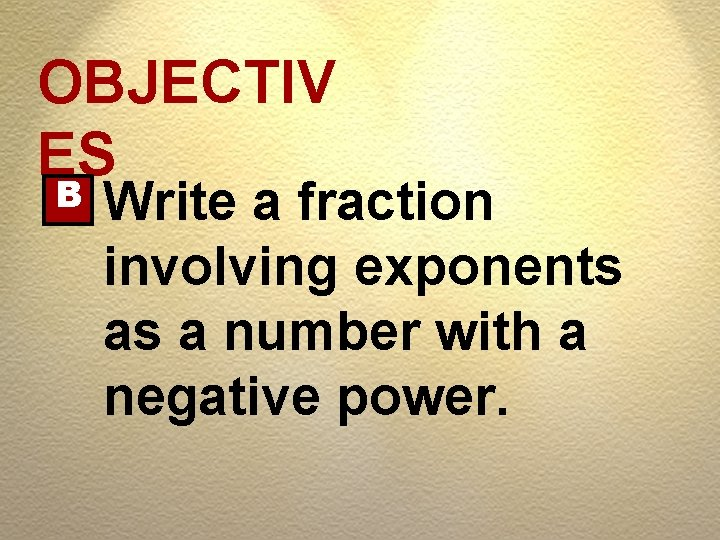OBJECTIV ES B Write a fraction involving exponents as a number with a negative power.OBJECTIV ES C Multiply and divide expressions involving negative exponents.RULES Zero Exponent Negative Exponent If n is a positive integer,RULES th n Power of a Quotient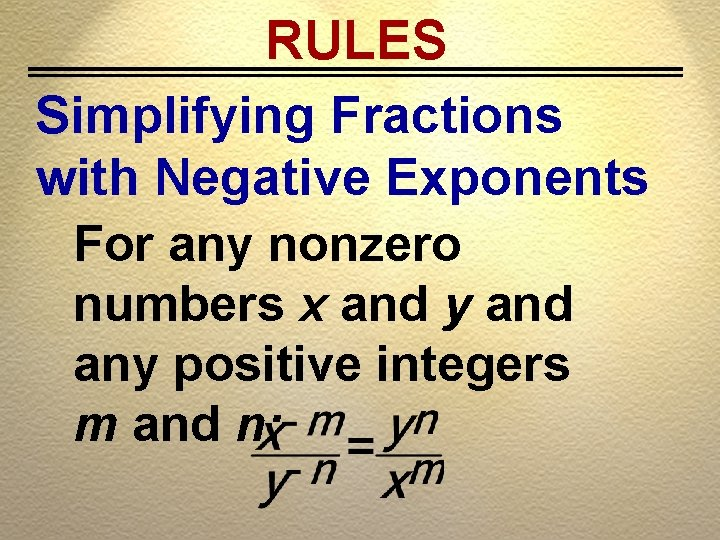RULES Simplifying Fractions with Negative Exponents For any nonzero numbers x and y and any positive integers m and n: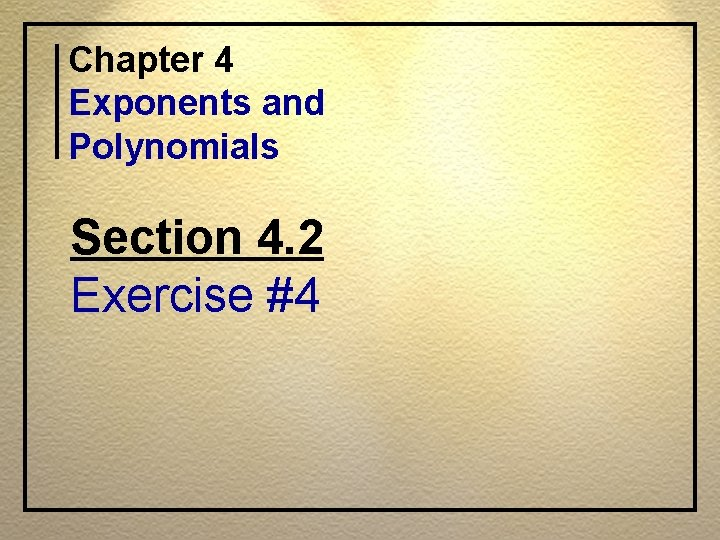Chapter 4 Exponents and Polynomials Section 4. 2 Exercise #4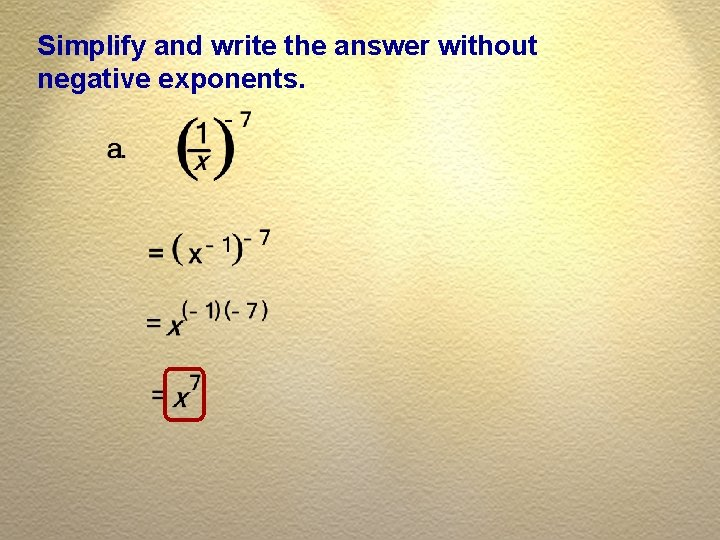Simplify and write the answer without negative exponents.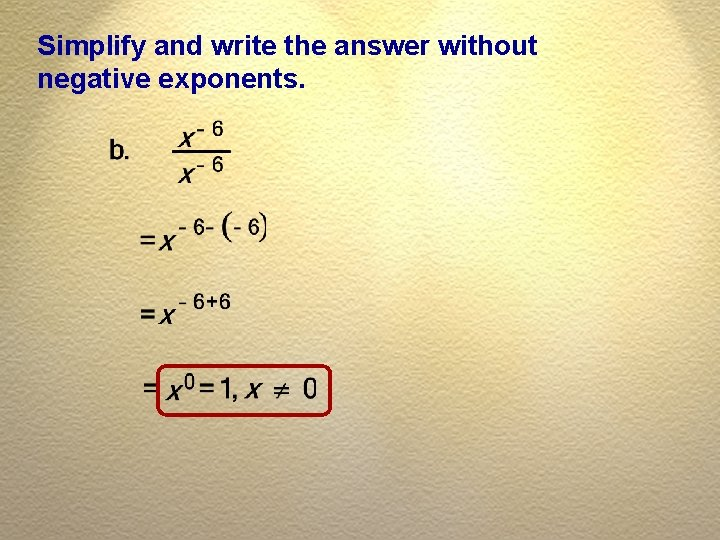Simplify and write the answer without negative exponents.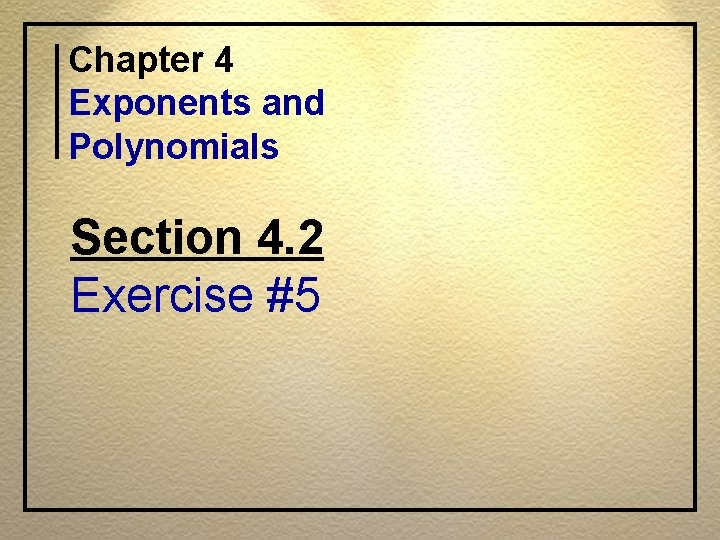Chapter 4 Exponents and Polynomials Section 4. 2 Exercise #5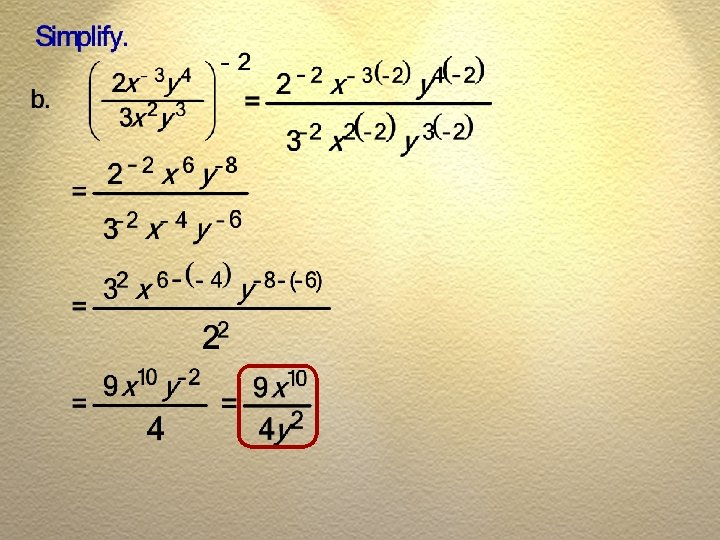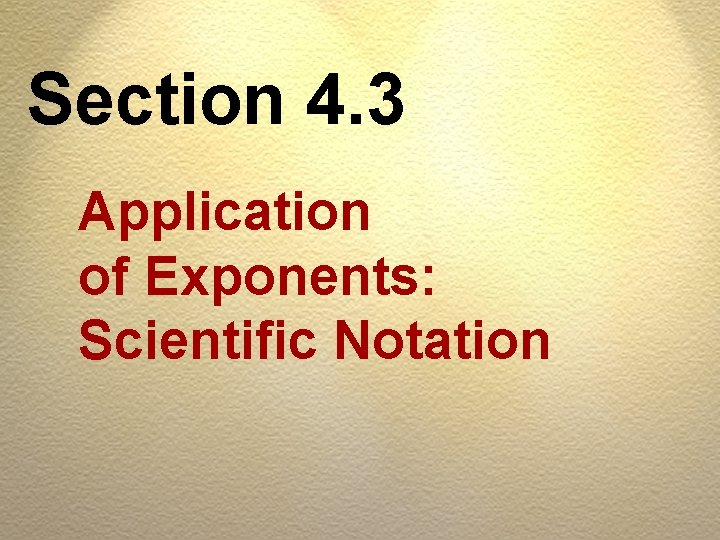Section 4. 3 Application of Exponents: Scientific NotationOBJECTIV ES A Write numbers in scientific notation.OBJECTIV ES B Multiply and divide numbers in scientific notation. C Solve applications.RULES A number in scientific notation is written as Where M is a number between 1 and 10 and n is an integer.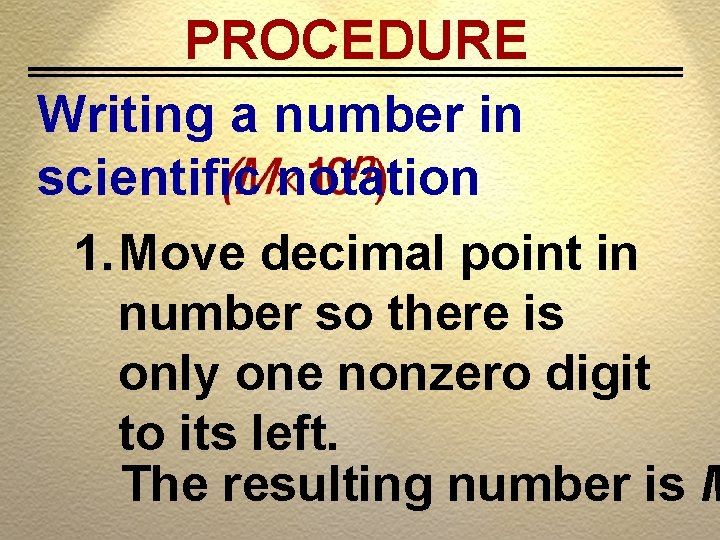PROCEDURE Writing a number in scientific notation 1. Move decimal point in number so there is only one nonzero digit to its left. The resulting number is MPROCEDURE Writing a number in scientific notation 2. If the decimal point is moved to the left, n is positive; If the decimal point is moved to the right, n is negative.PROCEDURE Writing a number in scientific notationPROCEDURE Multiplying using scientific notation 1. Multiply decimal parts first. Write result in scientific notation.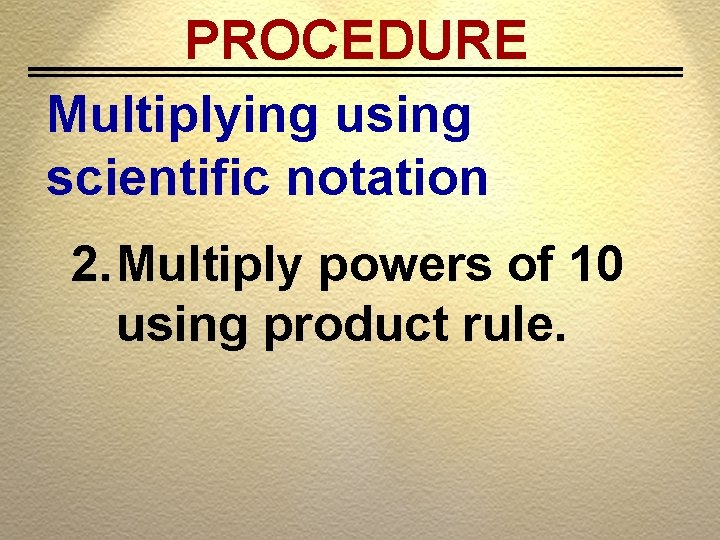PROCEDURE Multiplying using scientific notation 2. Multiply powers of 10 using product rule.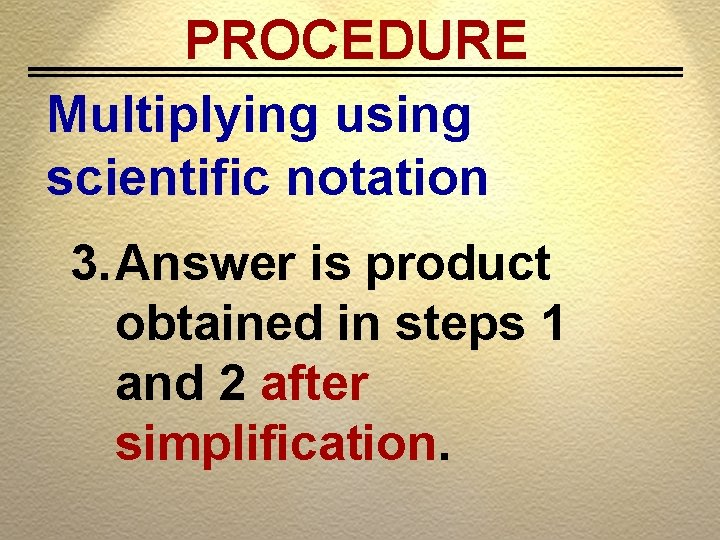PROCEDURE Multiplying using scientific notation 3. Answer is product obtained in steps 1 and 2 after simplification.Chapter 4 Exponents and Polynomials Section 4. 3 Exercise #6Write in scientific notation.Chapter 4 Exponents and Polynomials Section 4. 3 Exercise #7Perform the indicated operations.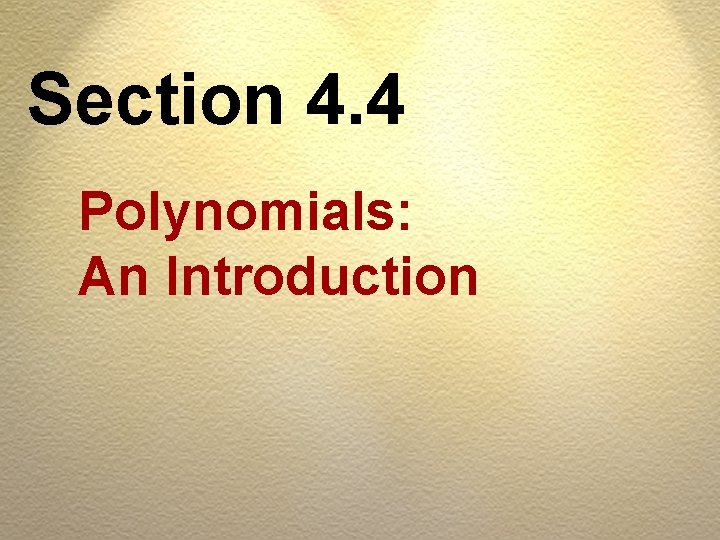Section 4. 4 Polynomials: An IntroductionOBJECTIV ES A Classify B polynomials. Find the degree of a polynomial.OBJECTIV ES C Write a polynomial in descending order. D Evaluate polynomials.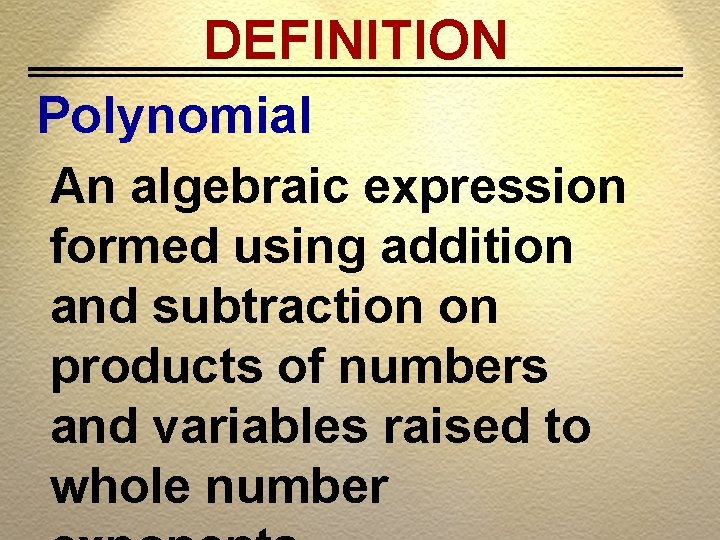DEFINITION Polynomial An algebraic expression formed using addition and subtraction on products of numbers and variables raised to whole number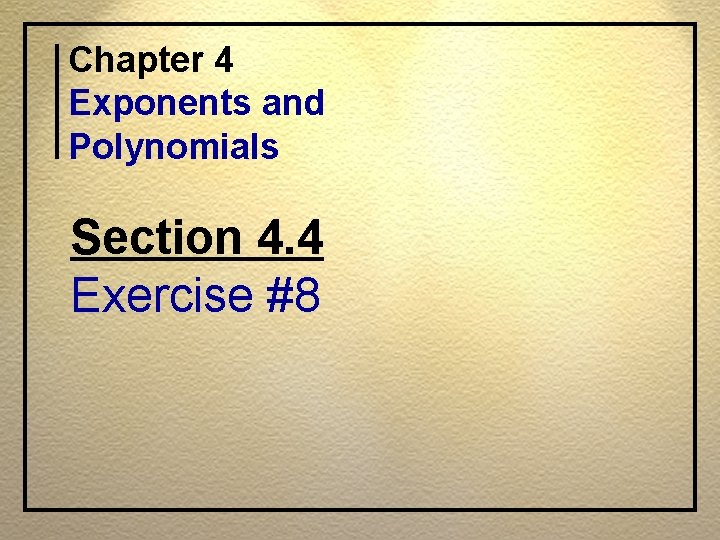Chapter 4 Exponents and Polynomials Section 4. 4 Exercise #8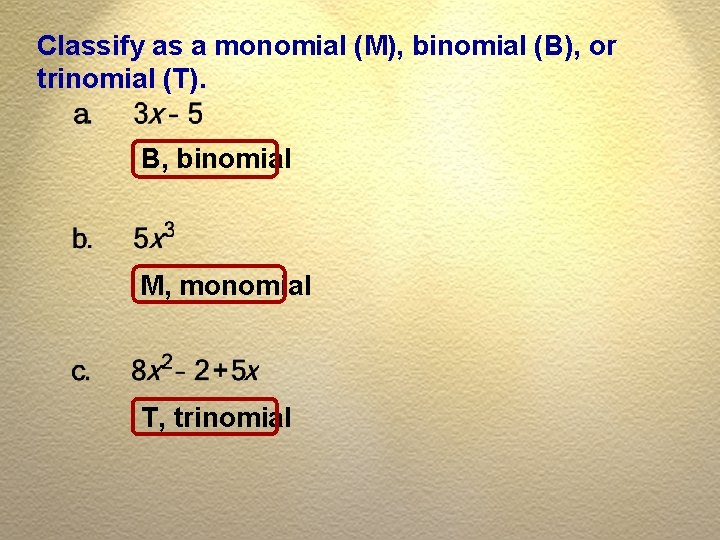Classify as a monomial (M), binomial (B), or trinomial (T). B, binomial M, monomial T, trinomial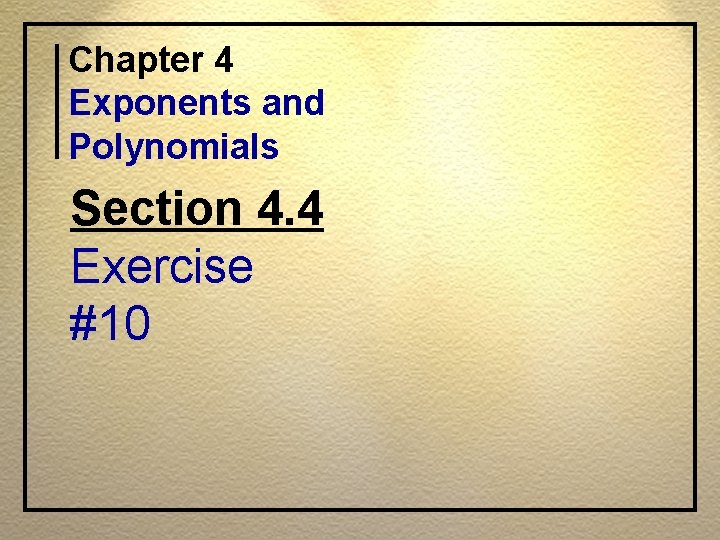Chapter 4 Exponents and Polynomials Section 4. 4 Exercise #10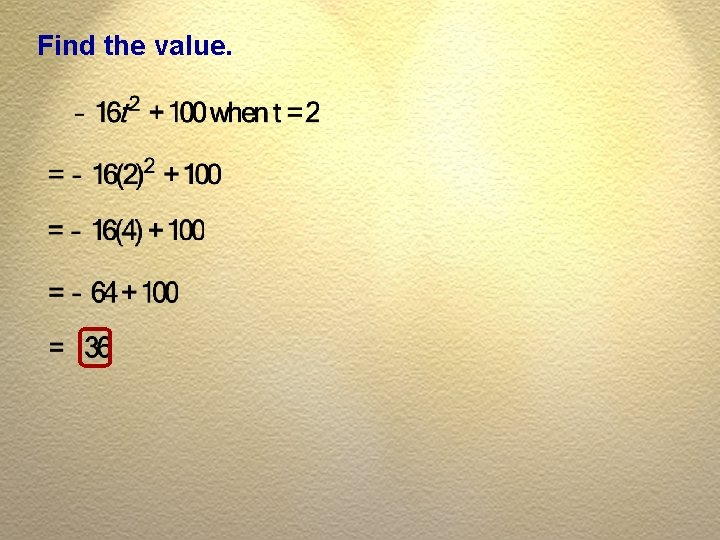Find the value.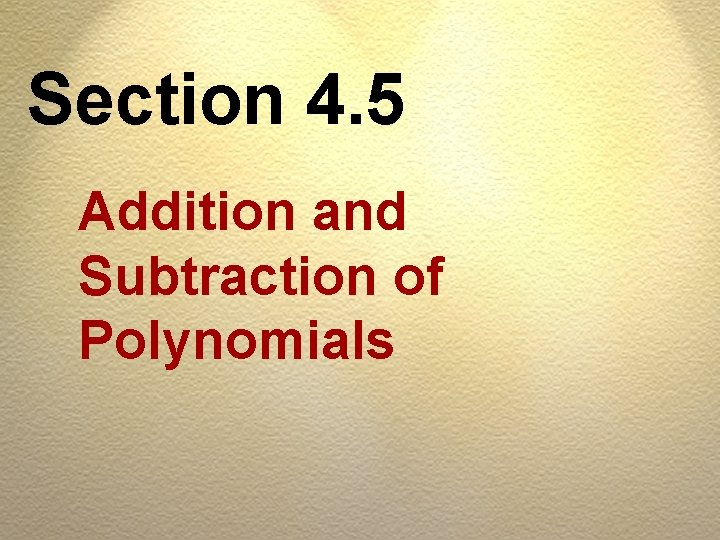Section 4. 5 Addition and Subtraction of PolynomialsOBJECTIV ES A Add polynomials. B Subtract polynomials.OBJECTIV ES C Find areas by adding polynomials. D Solve applications.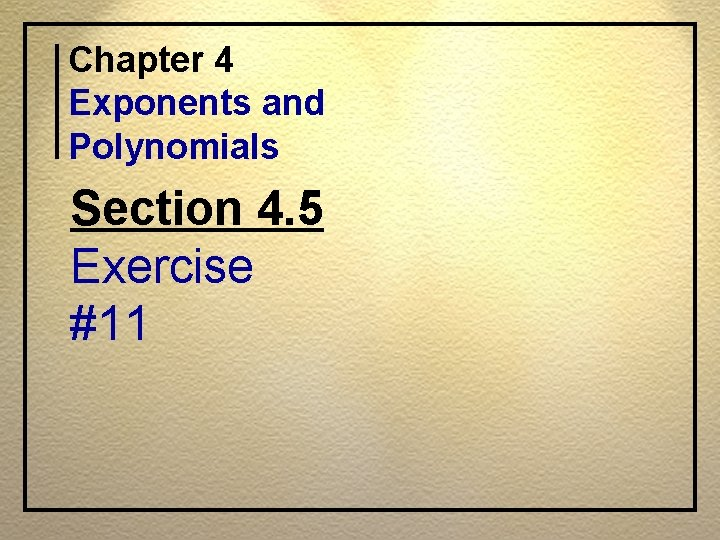Chapter 4 Exponents and Polynomials Section 4. 5 Exercise #11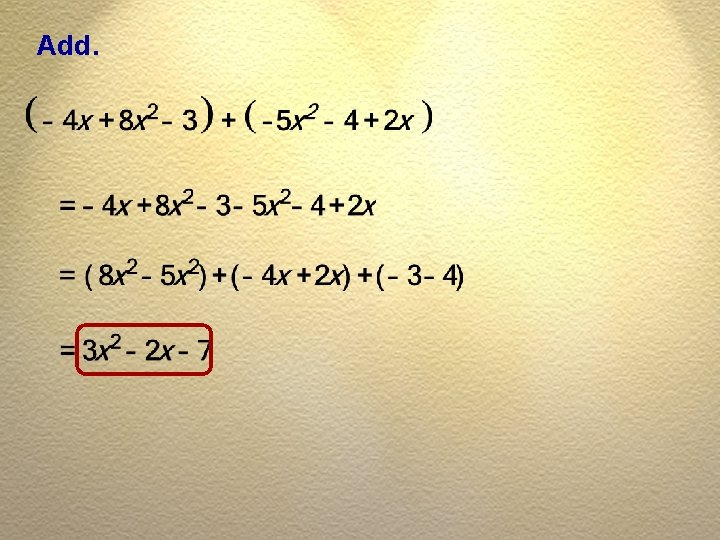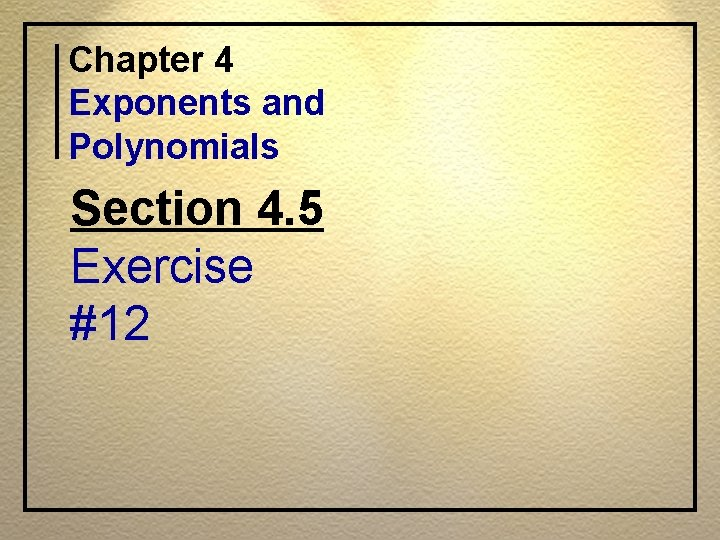Chapter 4 Exponents and Polynomials Section 4. 5 Exercise #12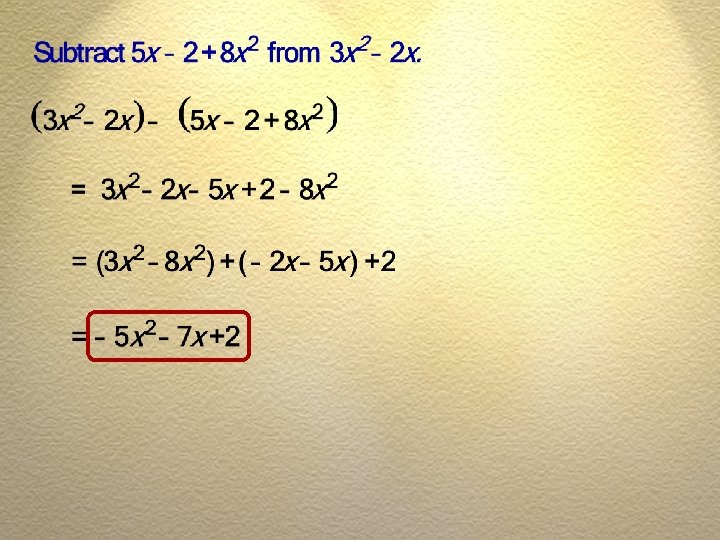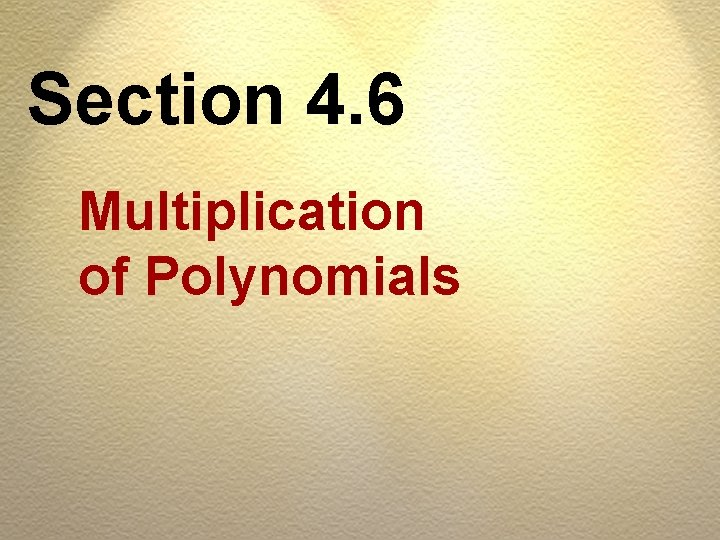Section 4. 6 Multiplication of Polynomials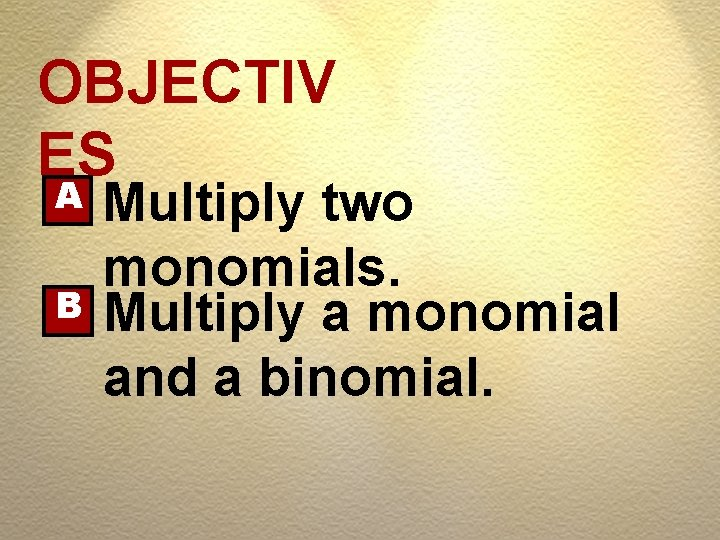OBJECTIV ES A B Multiply two monomials. Multiply a monomial and a binomial.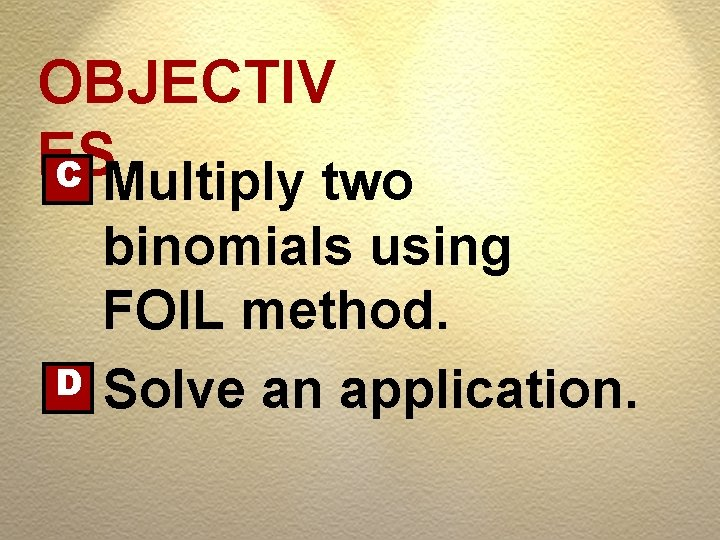OBJECTIV ES C Multiply two D binomials using FOIL method. Solve an application.PROCEDURE FOIL Method for Multiplying Binomials First terms multiplied first. Outer terms multiplied second. Inner terms multiplied third. Last terms multipliedChapter 4 Exponents and Polynomials Section 4. 6 Exercise #16F L O ISection 4. 7 Special Product of PolynomialsOBJECTIV ES Expand binomials of the form A B C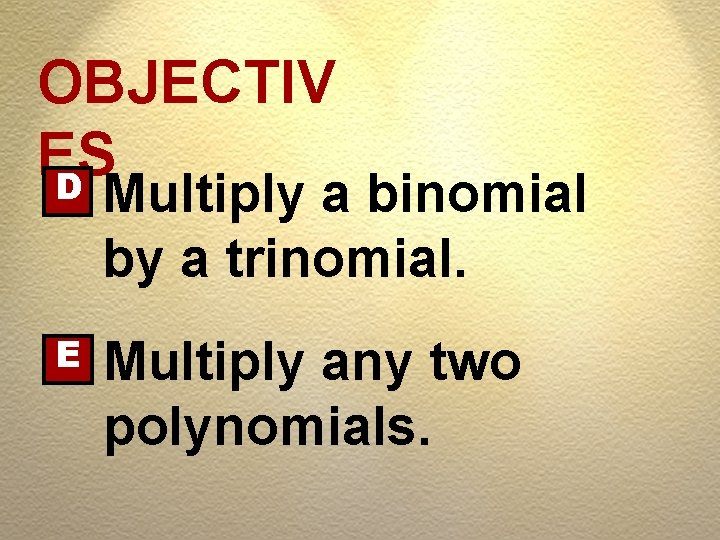OBJECTIV ES D Multiply a binomial by a trinomial. E Multiply any two polynomials.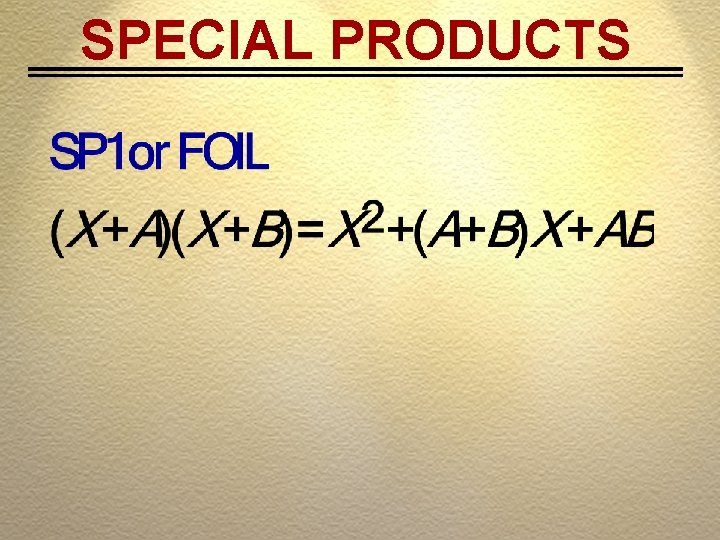SPECIAL PRODUCTS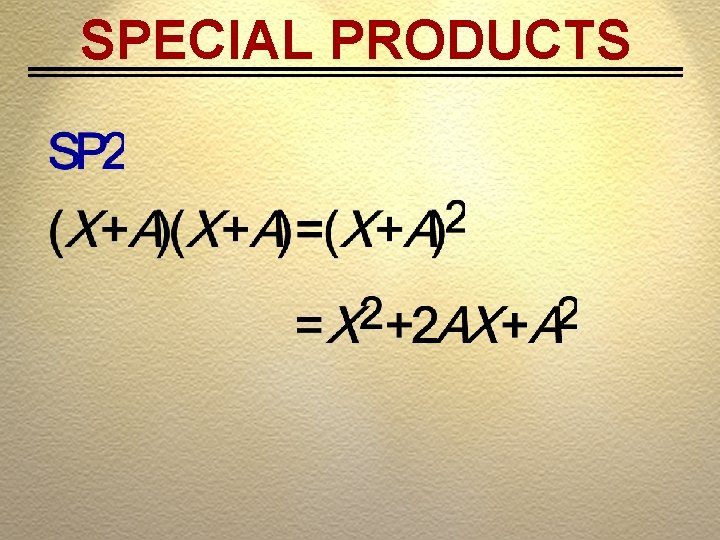SPECIAL PRODUCTS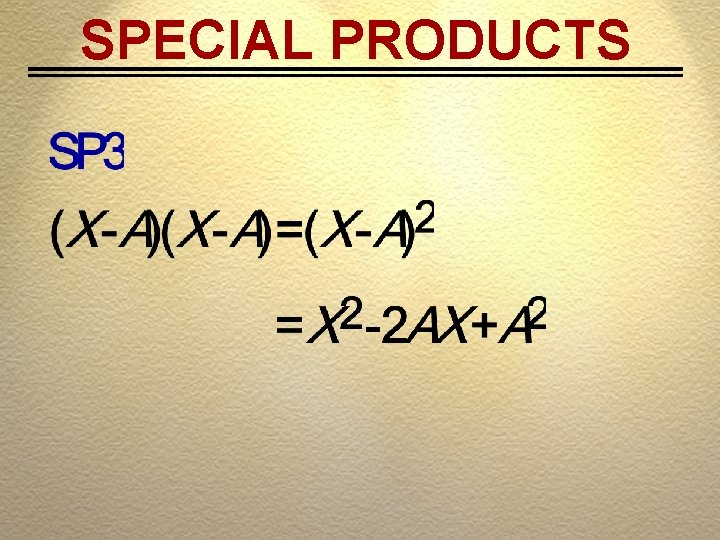SPECIAL PRODUCTS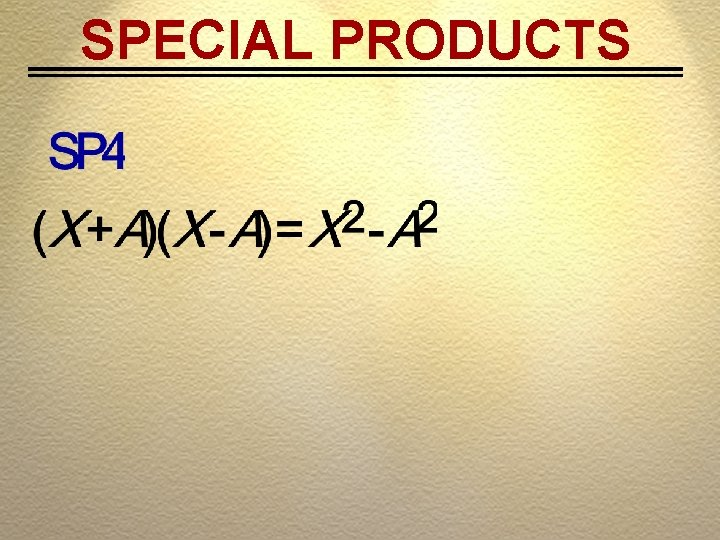SPECIAL PRODUCTS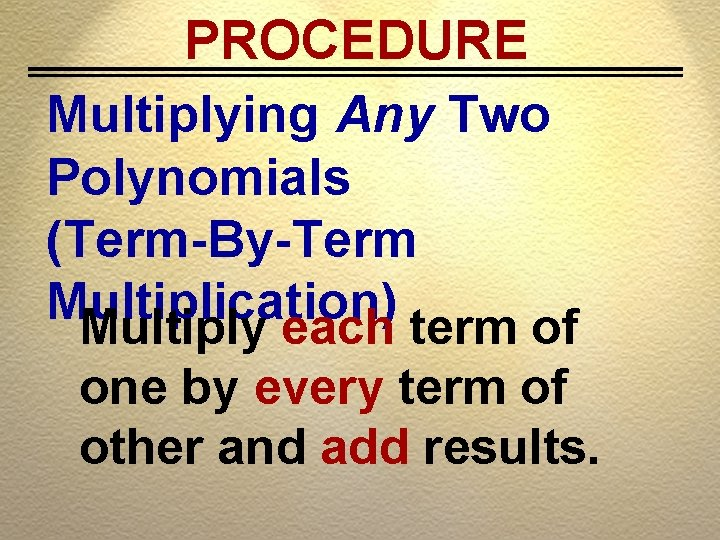PROCEDURE Multiplying Any Two Polynomials (Term-By-Term Multiplication) Multiply each term of one by every term of other and add results.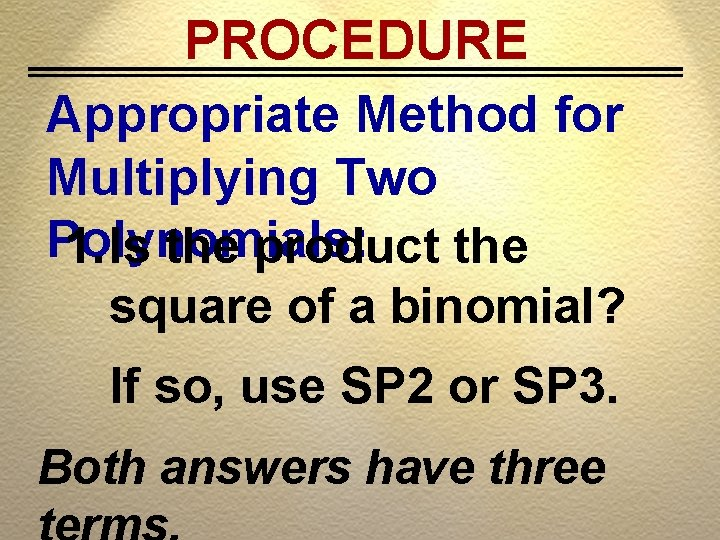PROCEDURE Appropriate Method for Multiplying Two Polynomials: 1. Is the product the square of a binomial? If so, use SP 2 or SP 3. Both answers have three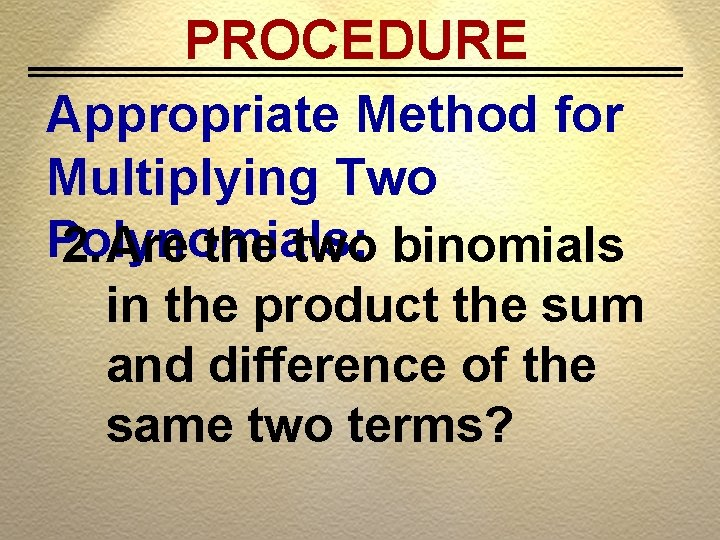PROCEDURE Appropriate Method for Multiplying Two Polynomials: 2. Are the two binomials in the product the sum and difference of the same two terms?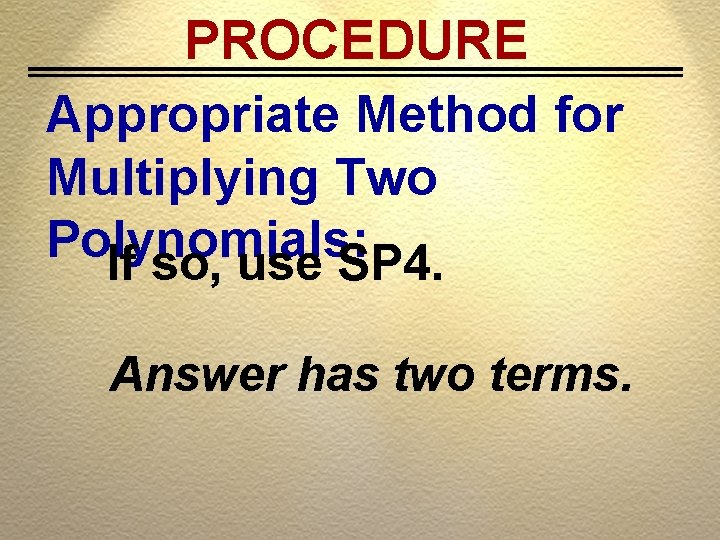PROCEDURE Appropriate Method for Multiplying Two Polynomials: If so, use SP 4. Answer has two terms.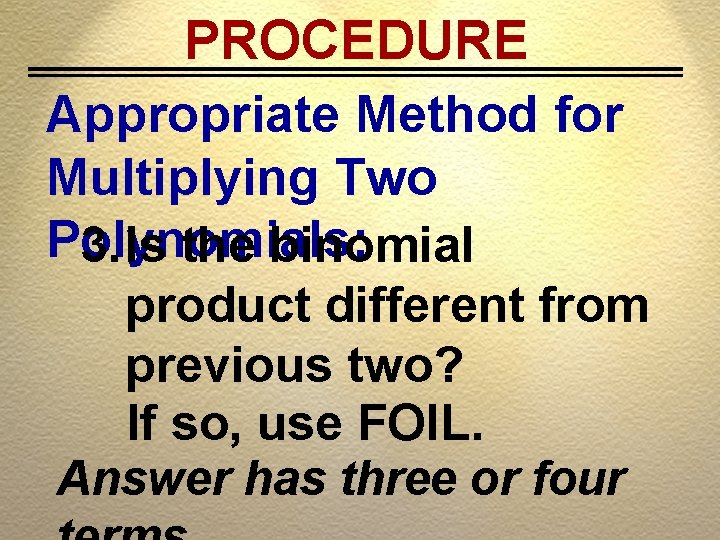PROCEDURE Appropriate Method for Multiplying Two Polynomials: 3. Is the binomial product different from previous two? If so, use FOIL. Answer has three or four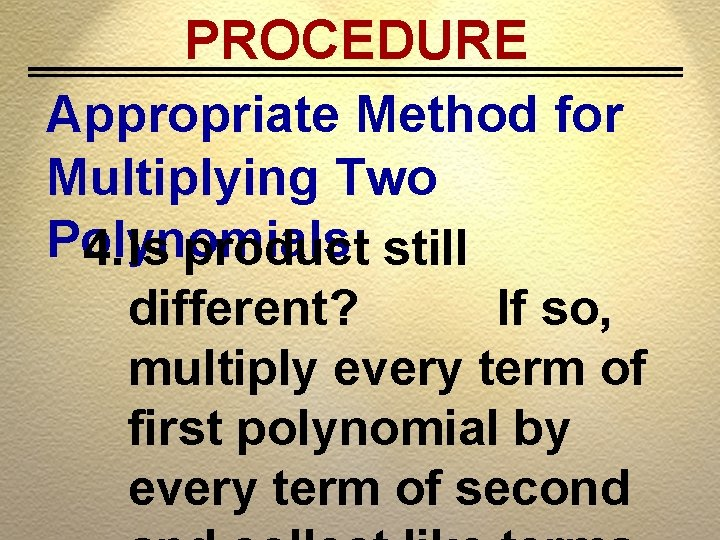PROCEDURE Appropriate Method for Multiplying Two Polynomials: 4. Is product still different? If so, multiply every term of first polynomial by every term of second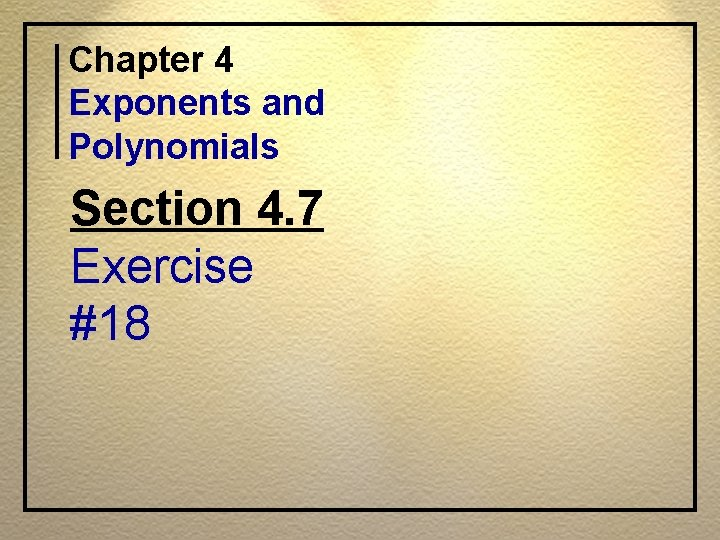Chapter 4 Exponents and Polynomials Section 4. 7 Exercise #18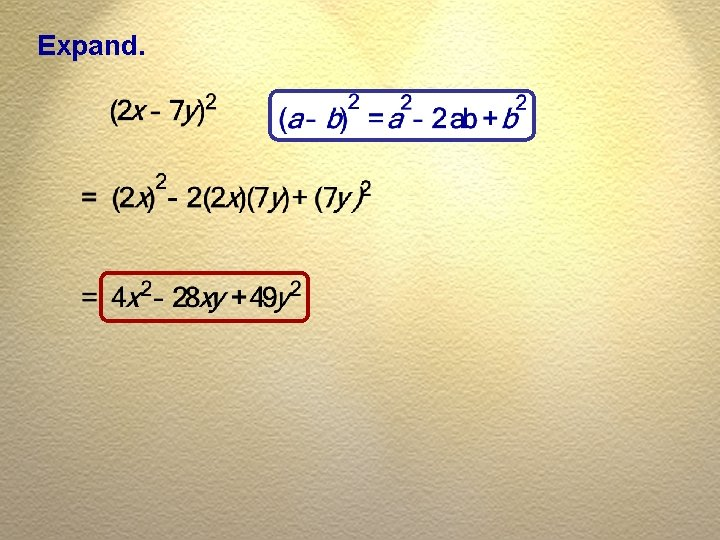Expand.Chapter 4 Exponents and Polynomials Section 4. 7 Exercise #19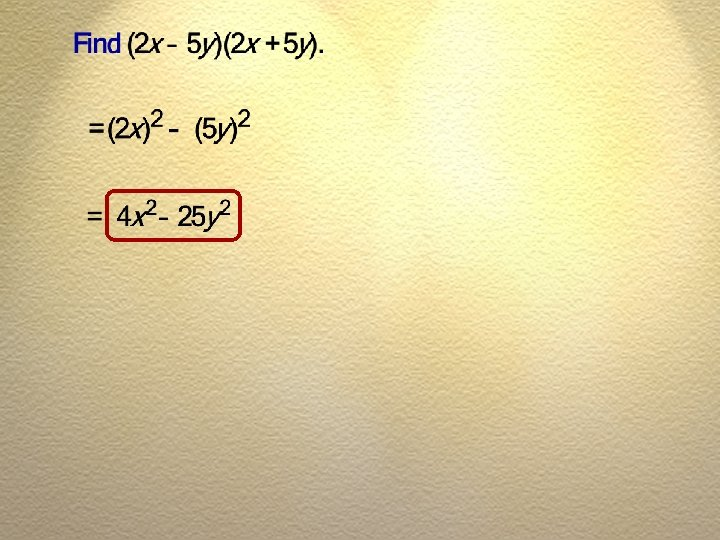Chapter 4 Exponents and Polynomials Section 4. 7 Exercise #20Find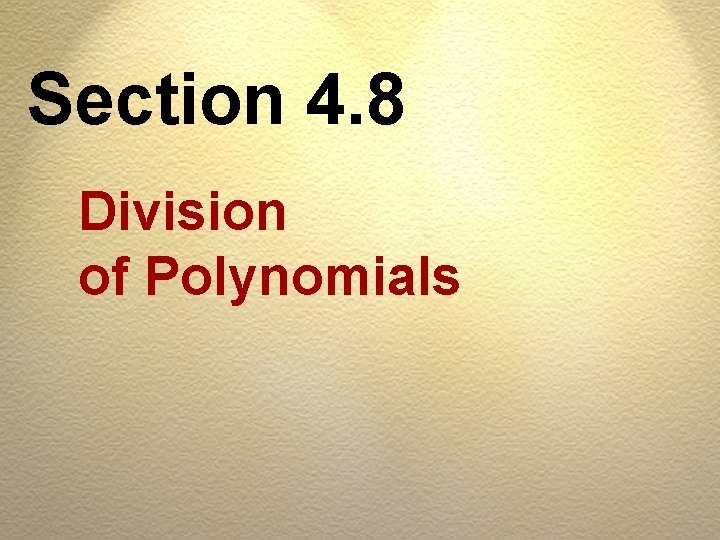Section 4. 8 Division of PolynomialsOBJECTIV ES A Divide a polynomial by a monomial. B Divide one polynomial by another polynomial.RULE To Divide A Polynomial By A Monomial Divide each term in polynomial by monomial.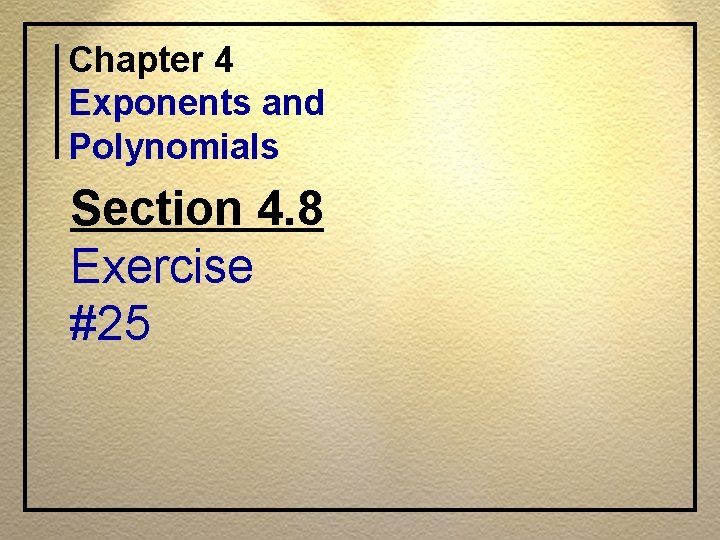Chapter 4 Exponents and Polynomials Section 4. 8 Exercise #25Divide.